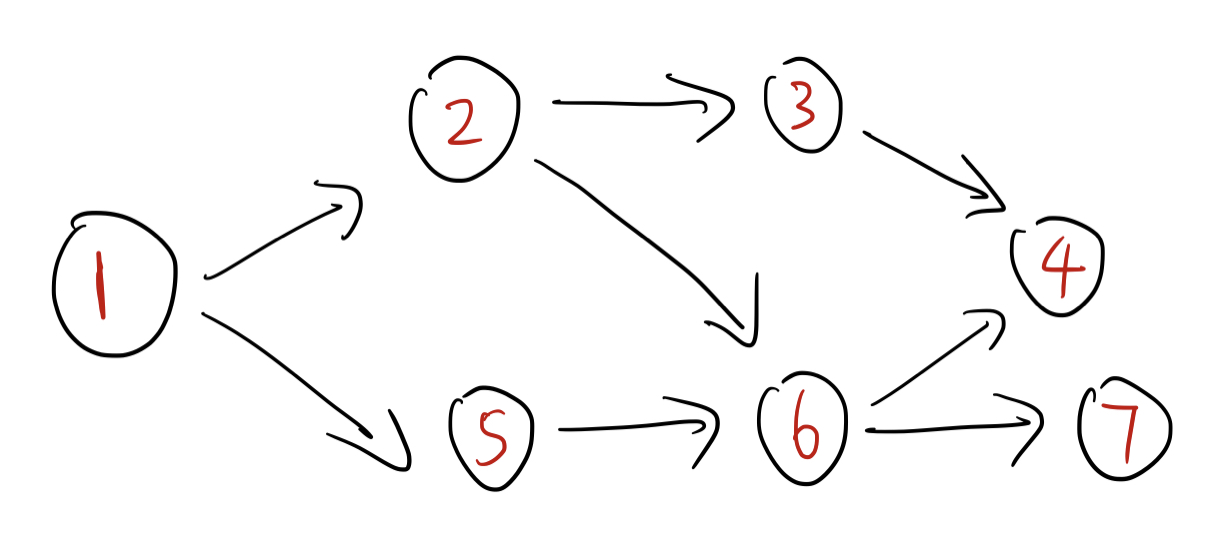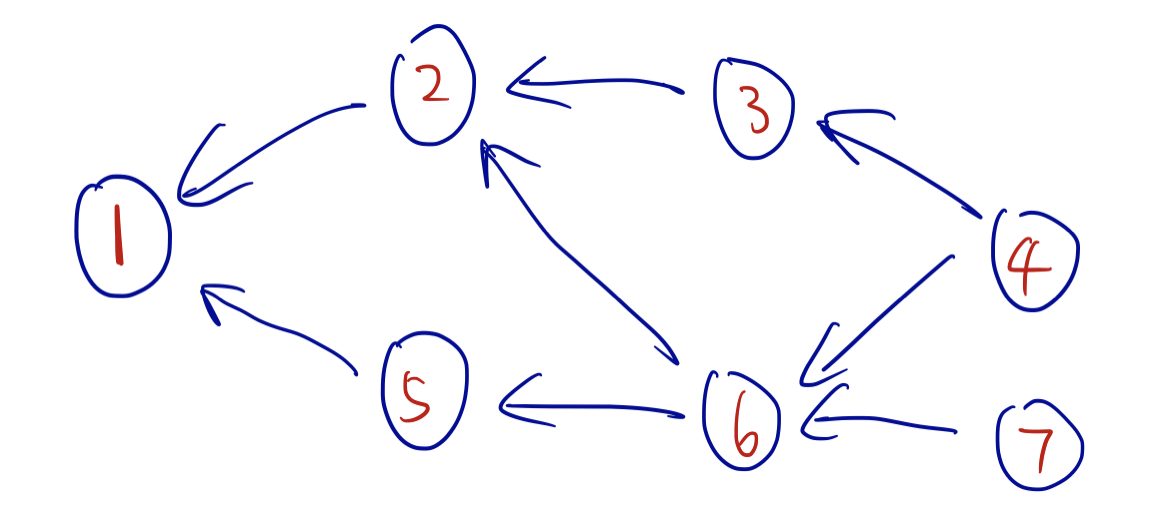## 定义

SCC（强连通分量）：一个极大的强连通子图。

• 缩点后得到的图一定是一个 DAG（有向无环图）

• DAG 有着很多优秀的性质，比如可以进行 拓扑排序，可以利用 拓扑排序进行 DP 等。

## Tarjan 求有向图的 SCC

### 算法流程

1. $dfn[u]$ ：DFS过程中，节点 $u$ 的编号（前序编号）。
2. $low[u]$ ：当前，在栈中的所有节点，以下两者的最小值：
1. $u$ 的子树中，所有节点 $v$ 的 $low[v]$ 最小值。
2. 从 $u$ 出发，经过一条 非树边 达到节点 $v$ 的 $dfn[v]$ 的最小值。
void dfs(int u) {
// ....
in[u] = 1;  // u 进栈
st[++tail] = u;  // 进栈
dfn[u] = low[u] = ++id;  // 前序编号
for (int e = head[u]; e; e = edges[e].nxt) {
int to = edges[e].to;
if (dfn[to] && in[to]) low[u] = min(low[u], dfn[to]);  // 需要在栈内
if (!dfn[to]) {
dfs(to);
low[u] = min(low[u], low[to]);
}
}
// ....


// from[u] 代表 u 所在的SCC编号，scc代表scc编号，sz[scc] 代表对应scc的大小
int dfn[maxn], low[maxn], id, from[maxn], scc = 0, sz[maxn];
bool in[maxn];  // instack or not
int st[maxn], tail = -1;
void dfs(int u) {
in[u] = 1;
st[++tail] = u;
dfn[u] = low[u] = ++id;
for (int e = head[u]; e; e = edges[e].nxt) {
int to = edges[e].to;
if (dfn[to] && in[to]) low[u] = min(low[u], dfn[to]);  // 要记得在栈内
if (!dfn[to]) {
dfs(to);
low[u] = min(low[u], low[to]);
}
}

if (dfn[u] == low[u]) {
from[u] = ++scc;
sz[scc] = 1;
while (tail >= 0 && st[tail] != u) {
int cur = st[tail];
from[cur] = from[u];
sz[scc]++;
tail--;
in[cur] = 0;  // 记得这里，将在栈中的标记去掉
}
tail--;
in[u] = 0;  // 记得这里，将在栈中的标记去掉
}
}


### 例题 洛谷P2341 [USACO03FALL][HAOI2006]受欢迎的牛 G

• 如果新图中有 $2$ 个明星，那么 明星 $1$ 存在到 明星 $2$ 的边，反之亦然。那么明星 $1,2$ 就属于同一个SCC，contradiction。

const int maxn = 1e4+5;
const int maxm = 5e4+10;

struct Edge {
int to, nxt;
} edges[maxm];
int head[maxn], ecnt = 1, n,m;

void addEdge(int u, int v) {
edges[ecnt] = e;
}

int dfn[maxn], low[maxn], id, from[maxn], scc = 0, sz[maxn];
bool in[maxn];  // instack or not
int st[maxn], tail = -1;
void dfs(int u) {
in[u] = 1;
st[++tail] = u;
dfn[u] = low[u] = ++id;
for (int e = head[u]; e; e = edges[e].nxt) {
int to = edges[e].to;
if (dfn[to] && in[to]) low[u] = min(low[u], dfn[to]);
if (!dfn[to]) {
dfs(to);
low[u] = min(low[u], low[to]);
}
}

if (dfn[u] == low[u]) {
from[u] = ++scc;
sz[scc] = 1;
while (tail >= 0 && st[tail] != u) {
int cur = st[tail];
from[cur] = from[u];
sz[scc]++;
tail--;
in[cur] = 0;
}
tail--;
in[u] = 0;
}
}

int deg[maxn];
void build() {
for (int u = 1; u <= n; u++) {
for (int e = head[u]; e; e = edges[e].nxt) {
int v = edges[e].to;
int fu = from[u], fv = from[v];
if (fv == fu) continue;  // 记得去掉自环
deg[fu]++;
}
}
}

void tarjan() {
for (int i = 1; i <= n; i++) {
if (!dfn[i]) dfs(i);
}
}

int main() {
cin >> n >> m;
for (int i = 1; i <= m; i++) {
int u,v; cin >> u >> v;
}
tarjan();
build();
int cnt = 0, ans;
for (int i = 1; i <= scc; i++) {
if (!deg[i]) cnt++, ans = i;
}
if (cnt > 1) cout << 0 << endl;
else cout << sz[ans] << endl;
}


## kosaraju 算法

kosaraju 算法本质上利用了 正反 $2$ 次DFS 求出一个图中的强连通分量。

### 算法流程

1. 建立原图 $G$，和一个反图 $G'$（将所有的边反过来）

2. 在原图 $G$ 上跑 DFS，回溯 的时候记录 ID。

3. 在反图 $G'$ 上跑 DFS，起始节点的顺序是 ID从大到小。每次 DFS 的 起始节点 就代表了一个新的SCC，DFS访问到的所有节点就是这个SCC。

### 感性理解### 模版题

const int maxn = 1e4+5;
const int maxm = 5e4+5;

struct Edge {
int to, nxt;
} edges[maxm], redges[maxm];

void addEdge(int u, int v) {
edges[ecnt++] = e;
}

void rev_addEdge(int u, int v) {
redges[recnt++] = e;
}

int id[maxn], idcnt = 0;
bool vis[maxn];
void dfs(int u) {
vis[u] = 1;
for (int e = head[u]; e; e = edges[e].nxt) {
int to = edges[e].to;
if (vis[to]) continue;  // 已经访问过了，忽略
dfs(to);
}
id[++idcnt] = u;  // 回溯的时候更新ID
}

int sz[maxn];
void dfs2(int u) {
sz[u] = 1;
for (int e = rhead[u]; e; e = redges[e].nxt) {
int to = redges[e].to;
if (sz[to]) continue;  // 已经访问过，忽略
dfs2(to);
sz[u] += sz[to];
}
}

int main() {
int n,m; cin >> n >> m;
for (int i = 1; i <= m; i++) {
int u,v; cin >> u >> v;
}

for (int i = 1; i <= n; i++) {
if (!vis[i]) dfs(i);
}

int ans = 0;
for (int i = n; i >= 1; i--) {
if (!sz[id[i]]) {  // 还没dfs过，说明这是一个新的SCC
dfs2(id[i]);
if (sz[id[i]] > 1)
ans++;
}
}
cout << ans << endl;
}


## 例题

### 例1 洛谷P3119 [USACO15JAN]Grass Cownoisseur G

1. 无环（仍然是一个DAG）
2. $G_1 \rightarrow G_2$ 只能发生一次。（从 $G_2$ 无法返回 $G_1$）

• 注：最长路不能用 dijkstra，只能用 SPFA。复杂度最坏 $O(nm)$

• 注2：对于 DAG 而言，求最长路也可以直接用 拓扑排序 + DP。复杂度 $O(n+m)$，在DAG中，可以完美替代 SPFA

• 注3：需要特判一下原图是不是一个SCC（一般这种题都要特判一下）。如果是，直接输出答案 $n$。

#include <bits/stdc++.h>
using namespace std;
const int maxn = 1e5+5;

struct Edge {
int to,nxt;
} edges[maxn];
int head[maxn], ecnt = 1, id = 0, scc = 0, from[maxn], sz[maxn], st[maxn], tail = -1, n, m, dfn[maxn], low[maxn];
bool in[maxn];

void addEdge(int u, int v) {
edges[ecnt] = e;
}

void dfs(int u) {
dfn[u] = low[u] = ++id;
in[u] = 1;
st[++tail] = u;
for (int e = head[u]; e; e = edges[e].nxt) {
int to = edges[e].to;
if (dfn[to] && in[to]) {
low[u] = min(low[u], dfn[to]);
}
if (!dfn[to]) {
dfs(to);
low[u] = min(low[u], low[to]);
}
}

if (dfn[u] == low[u]) {
from[u] = ++scc;
sz[scc] = 1;
while (st[tail] != u) {
int cur = st[tail--];
from[cur] = from[u];
sz[scc]++;
in[cur] = 0;
}
tail--;
in[u] = 0;
}
}

void tarjan() {
for (int i = 1; i <= n; i++) {
if (!dfn[i]) dfs(i);
}
}

struct New_Edge {
int to, nxt, w;
} new_edges[maxn<<2];

void new_addEdge(int u, int v, int w) {
New_Edge e = {v, new_head[u], w};
new_edges[new_ecnt++] = e;
}

void build() {
for (int u = 1; u <= n; u++) {
for (int e = head[u]; e; e = edges[e].nxt) {
int v = edges[e].to;
int fu = from[u], fv = from[v];
if (fu == fv) continue;   // 注意判重
}
}
}

int d[maxn<<1];  // 因为复制了一份，记得开2倍大小
bool inq[maxn<<1];
queue<int> q;
void spfa() {
q.push(from);
inq[from] = 1;  // 注意是 from
while (!q.empty()) {
int cur = q.front(); q.pop();
inq[cur] = 0;
for (int e = new_head[cur]; e; e = new_edges[e].nxt) {
int to = new_edges[e].to, w = new_edges[e].w;
if (d[cur] + w > d[to]) {  // 无论是否 inq[] 都要更新
d[to] = d[cur] + w;
if (!inq[to])
q.push(to);
inq[to] = 1;
}
}
}
}

int main() {
cin >> n >> m;
for (int i = 1; i <= m; i++) {
int u,v; cin >> u >> v;
}
tarjan();
build();
spfa();

int ans = d[from + scc];  // 注意是 from
if (scc == 1) ans = n;  // 需要特判一下整个图是否为强连通分量
cout << ans << endl;
}



void solve() {
tail = -1;
fill(dp, dp+2*scc+1, -1e9);  // 注意赋值为 -inf，因为我们只关心从 from 出发的部分
dp[from] = 0;

for (int u = 1; u <= 2*scc; u++) {
if (!ind[u]) st[++tail] = u;
}
while (tail >= 0) {
int cur = st[tail--];
for (int e = new_head[cur]; e; e = new_edges[e].nxt) {
int to = new_edges[e].to, w = new_edges[e].w;
dp[to] = max(dp[to], dp[cur] + w);
ind[to]--;
if (!ind[to]) st[++tail] = to;
}
}
}

int main() {
cin >> n >> m;
for (int i = 1; i <= m; i++) {
int u,v; cin >> u >> v;